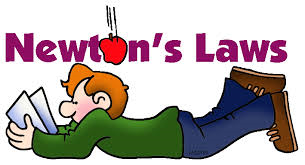## 7th GRADE SCIENCE : Article 17 : Newton's laws.NEWTONS LAWS.

* Activity 1.21   :   How a plane flies and forces involved.                          ( 10/2/17   Monday )

1.21.a    Plane reaction force reading and questions.    ( 10 points ).

1.21.b    Reaction force engine.   ( 10 points ).

* Activity 1.22   :  First contact to Newtons laws.                                            ( 10/3/17   Tuesday )

- Take a tablet from the chart-trolley and go to the following link to do this task.

1.22.a    First contact with Newtons laws.         ( 10 points ).

- Once you have looked this link up, answer the following questions :

- Who was Isaac Newton?.

- When did he live? Which century?.  Where?.

- What did he do?.

- How many motion laws did he sketch out?.

- What kind of scientist he was?.

- What another issues about science did he do?.

- What is the first Newtons law?.

- Tell a situation or example in which the first law is involved.

* Activity 1.23   :  Second Newtons law (Motion).                                ( 10/4/17   Wednesday )

answer the following questions :                                ( 10 points ).

a0)  Who wrote the second Newton's law?.

a1)  What is the difference between the first and the second Newtons law?.

a2) Write and explain what the second Newtons law says about forces and motion.

b) What is the mathematical equation for the second Newtons law?.

c) Tell an example in which the second Newtons law is involved.

d) Look this example (car)  up, later do the following similar exercise at your workbook:

Mikes car, which weighs 2,000 kg, is out of gas. Mike is trying to push the car to a gas station, and he makes the car go 0.1 m/s/s. Using Newtons Second Law, you can compute how much force Mike is applying to the car.

1.23.b   Go to the following LINK and summarize , using your own words, the most important that is said about the second Newtons law.       ( 5 points ).

1.23.c  At the following LINK you can make a test and check what you know about the second Newtons law after having done these activities.  ( 5 points ).

* Activity    :  No school today.                                                           ( 10/5/17   Thursday )

NO SCHOOL.

* Activity   :  No school today.                                                             ( 10/6/17   Friday )

NO SCHOOL.

Comentarios  Ir a formulario